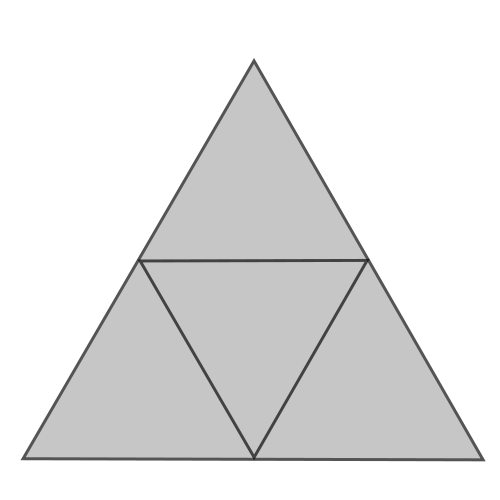# Triangles in Triangles in Triangles...

Probability Level 4What is the largest natural number $N$ such that we can NOT subdivide an equilateral triangle into $N$, not necessarily congruent, equilateral triangles?

Examples:

• We CAN subdivide an equilateral triangle into 4 equilateral triangles like in the image above.
• But we can NOT subdivide an equilateral triangle into 2 (possibly different) equilateral triangles.
×